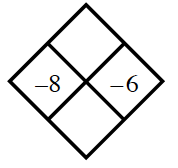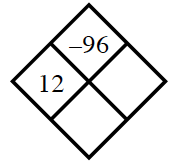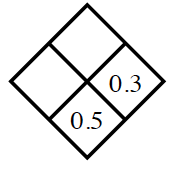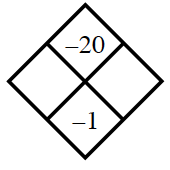### Home > CC2MN > Chapter 4 > Lesson 4.3.1 > Problem4-83

4-83.

Copy and complete each of the Diamond Problems below. The pattern used in the Diamond Problems is shown at right.1.To find $xy$, multiply $-8$ by $-6$.
$(-8)(-6)=48$

To find $x+y$, add $-8$ to $-6$.
$-8+(-6)=-14$

Top: $48$
Bottom: $-14$

1.Divide $-96$ by $12$ to find $y$.
Then use $x$ and $y$ to
find $x+y$.

1.$x=0.5-0.3$
Use $x$ and $0.3$ to find $xy$.

Top: $0.06$
Left: $0.2$

1.Make a list of the factors of $-20$.
$x$ and $y$ will have an absolute difference of $1$, with the larger number being negative.